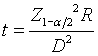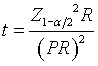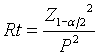# Confidence intervals for count data

## 2007-03-22

If you have data involving counts, you have several options for computing confidence intervals. All of these approaches rely on approximations to the Poisson distribution or to relationships involving the Poisson distribution and other important distributions. I want to summarize some of these approaches.

It may help to review the definition of the Poisson distribution.

### Confidence interval for a count variable

Suppose you have a single count variable that you believe comes from a Poisson distribution with mean pi. You want to compute a 95% confidence interval for pi.

A simple approach is to assume that the Poisson distribution can be approximated by a normal distribution with the same mean and standard deviation. If you have a count, C, that you believe comes from a Poisson distribution, then a confidence interval for the mean of this Poisson distribution isThis is a rather unusual situation in that most confidence intervals require a sample of more than just one value. But because a single parameter is both the mean and the standard deviation of the Poisson distribution, we can still compute a confidence interval.

Suppose you observe a count of 20 that represents a single observation from a Poisson distribution with unknown parameter <U+03BB>. You want a 95% confidence interval for <U+03BB>. The width of the 95% confidence interval would be 1.96*v20 = 8.8, so the confidence interval would range from 11.2 to 28.8.

The normal approximation is reasonable as long as <U+03BB> is large. Different sources quote different values for “large”, ranging from 5 to 20.

### Confidence interval for a rate variable

Quite often, you are interested in computing not a count, but a rate. A rate is simply a count divided by a measure of time or area. If you count a number of events C over a time period t, it is frequently useful to consider the counts to be distributed as a Poisson random variable with parameter pi t. This notation allows you flexibility in handling counts over varying time ranges. So a count over a 2.5 hour period would be Poisson with mean 2.5 pi, and a count of the same type of event over a 4.5 hour period would be Poisson with mean 4.5<U+03BB>.

If you computed a rate R = C/t, that rate would be a good estimate for pi. A 95% confidence interval for the expected rate isThis formula also relies on the normal approximation. Suppose you counted 61 events over a seven day period and computed a rate of 61/7 = 8.7 events per day. The 95% confidence interval would have a width of 1.96*v8.7/7 = 2.2. So the confidence interval would range from 6.5 to 10.9.

### Confidence interval for an average of several count variables

A very closely related problem is computing the average of a sample of counts. Suppose you collect the following data: C~1~, C~2~, …, C~n~.

Suppose that each count is independent and identically distributed Poisson variable with a common parameter <U+03BB>. The sum of those n counts is also distributed as a Poisson variable, but the parameter is n<U+03BB>. The average is a good estimate of <U+03BB>. A 95% confidence interval isNote that this formulas is effectively the same as the formula for the confidence interval for a rate if you let t=n.

With a sample of n counts, you could also compute a standard deviation using the traditional formulas. You should feel more comfortable with the assumption of a Poisson distribution if the sample standard deviation is close to### Justifying a sample size

Suppose you want to justify the sample size for a study whose primary goal is to estimate a rate. How would you do this?

One way is to get a confidence interval for the rate that has a sufficient amount of precision. If you want the confidence interval to have a width of no more than +/-D, then you need to solve the equationfor t, which produces the following equation.In order to calculate t, you need an estimate of R. You don’t know R, of course, but you probably have a rough idea of how large it would be. Suppose you are trying to estimate a rate that is likely to be larger than 4 per week, but smaller than 10 per week. You want to estimate this rate with a 95% confidence interval with a precision of plus/minus 2. If the rate is 4, then the sample time is 3.8 weeks (27 days). If the rate is 10, then the sample time is 15.2 weeks (106.4 days)

Suppose you wanted to pick a sample size so that the relative precision of the confidence interval was sufficiently small. Set the desired width of the confidence interval to PR where P is a proportion. Substitute PR for D in the above equation to getwhich simplifies toNote that Rt represents a count of the number of events across the full time range t. Suppose we wanted to have a 95% confidence interval for a rate with a width of plus/minus 0.4 times the rate., the relative precision of the confidence interval is a function not of the r. Substitute the value of P=0.4 in the above equation to get Rt=24. So we have to sample for a sufficient time frame to accumulate at least 24 events. If you wanted the 95% confidence interval to have a width of plus/minus 0.25 times the rate, you would have to sample for a sufficient time frame to in order to accumulate at least 62 events.

### Further work

In a later weblog entry, I want to outline the formula for an exact confidence interval that relies on the relationship between the cumulative sum of Poisson probabilities and the Chi-square or Gamma distributions. I also want to discuss some of the confidence intervals involving the comparison of two Poisson distributed counts.

Here is a web page which outlines additional details about these and other confidence intervals.

Standardized Incidence Ratio and Confidence Limits. Available in [html format][sse1]

You can find an earlier version of this page on my old website.